# 一致性 Hash 算法学习（分布式或均衡算法）

## 场景引入：

hash(object)%N

1） 某个 cache 服务器 m down 掉了（在实际应用中必须要考虑这种情况），这样所有映射到 cache m 的对象都会失效，怎么办，需要把 cache m 从 cache 中移除，这时候 cache 是 N-1 台，映射公式变成了 hash(object)%(N-1) ；

2） 由于访问加重，需要添加 cache ，这时候 cache 是 N+1 台，映射公式变成了 hash(object)%(N+1) ；

1） 和 2） 意味着什么？这意味着无论是添加或移除 cache 服务器，突然之间几乎所有的 cache 都失效了。对于服务器而言，这是一场灾难，洪水般的访问都会直接冲向后台服务器；

## hash 算法和单调性

Hash 算法的一个衡量指标是单调性（ Monotonicity ），定义如下：

单调性是指如果已经有一些内容通过哈希分派到了相应的缓冲中，又有新的缓冲加入到系统中。哈希的结果应能够保证原有已分配的内容可以被映射到新的缓冲中去，而不会被映射到旧的缓冲集合中的其他缓冲区。

## 一致性 Hash 算法原理：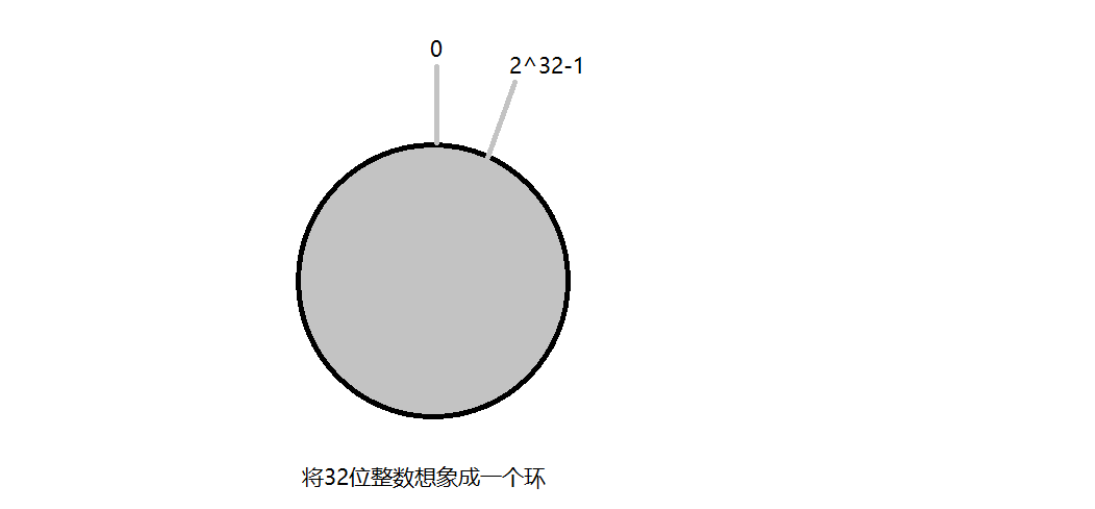key1 = hash(object1);
key2 = hash(object2);
key3 = hash(object3);
key4 = hash(object4);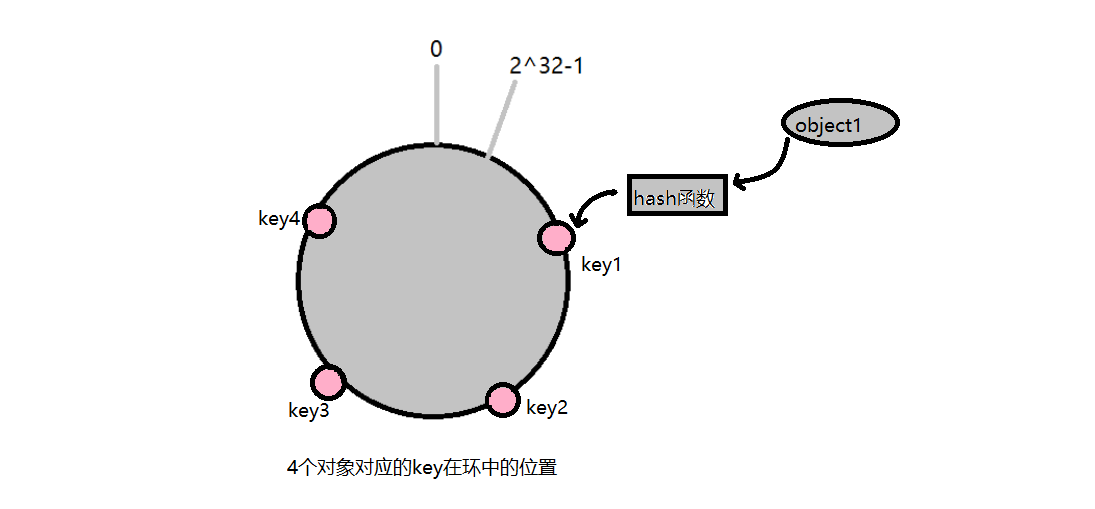keyA = hash(cacheA);
keyB = hash(cacheB);
keyC = hash(cacheC);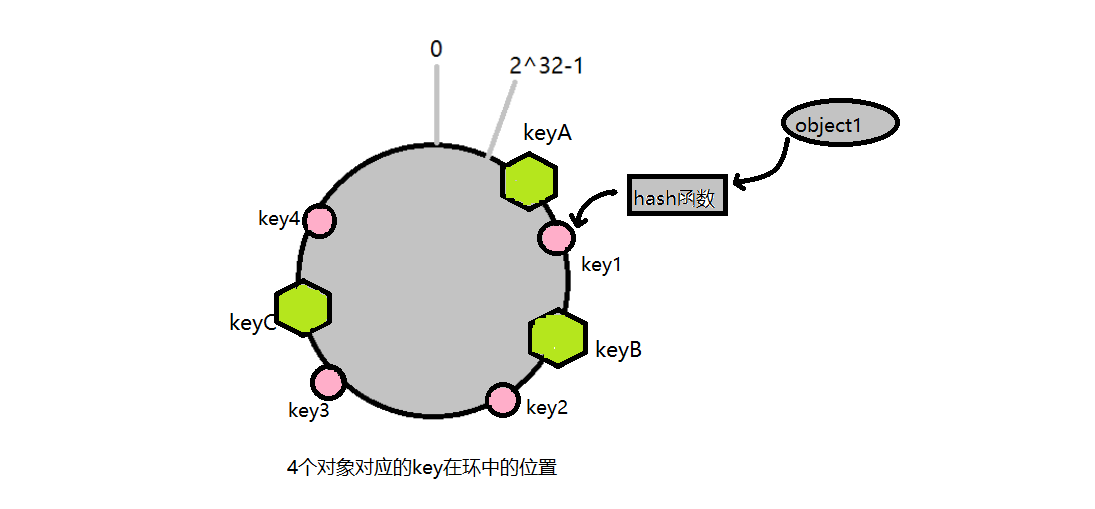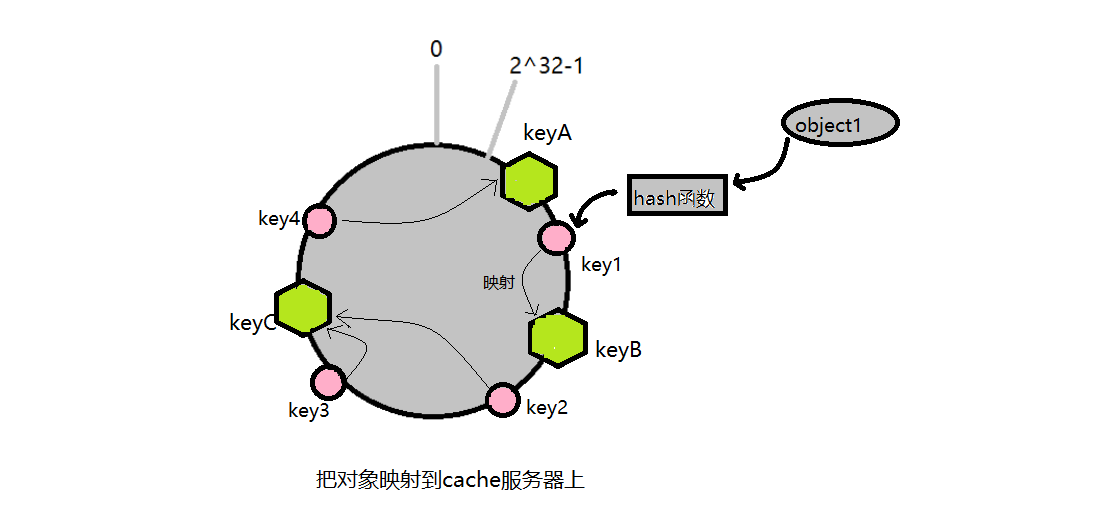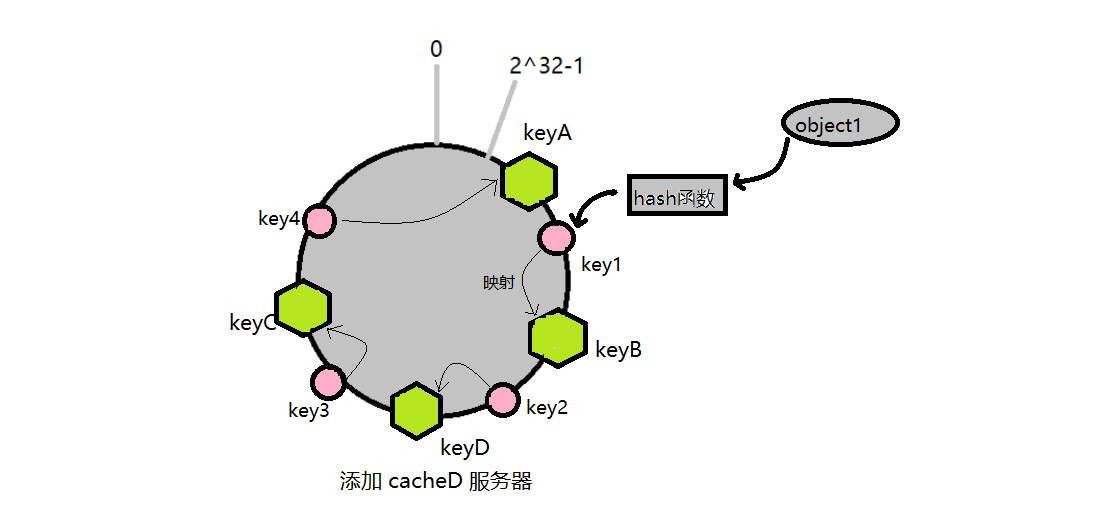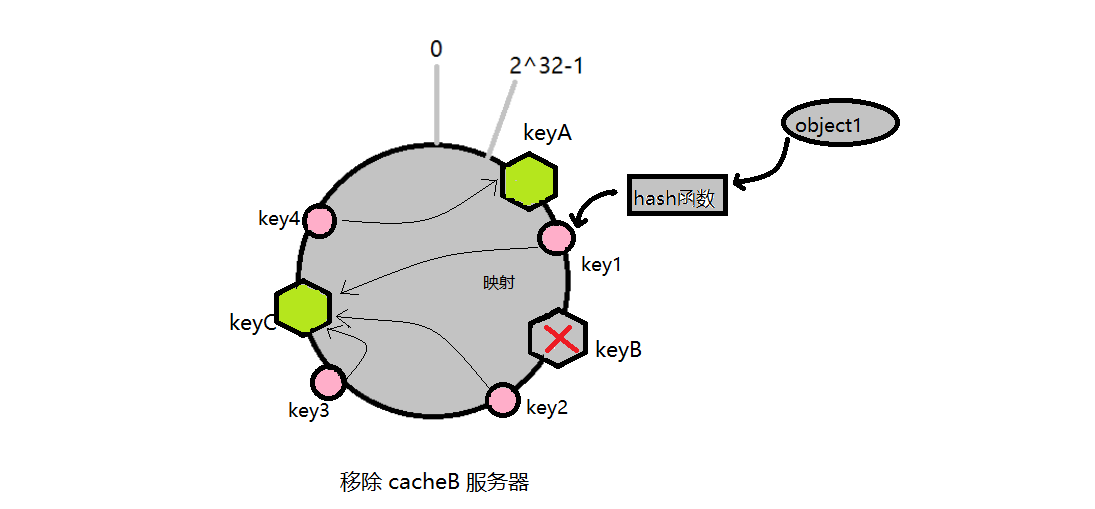## 平衡性与虚拟节点：

平衡性是指哈希的结果能够尽可能分布到所有的缓冲中去，这样可以使得所有的缓冲空间都得到利用。

hash 算法并不是保证绝对的平衡，如果 cache 较少的话，对象并不能被均匀的映射到 cache 上，比如在上面的例子中，仅部署 cache A 和 cache C 的情况下，在 4 个对象中， cache A 仅存储了 object1 ，而 cache C 则存储了 object2 、 object3 和 object4 ；分布是很不均衡的。

“虚拟节点”（ virtual node ）是实际节点在 hash 空间的复制品（ replica ），一实际个节点对应了若干个“虚拟节点”，这个对应个数也成为“复制个数”，“虚拟节点”在 hash 空间中以 hash 值排列。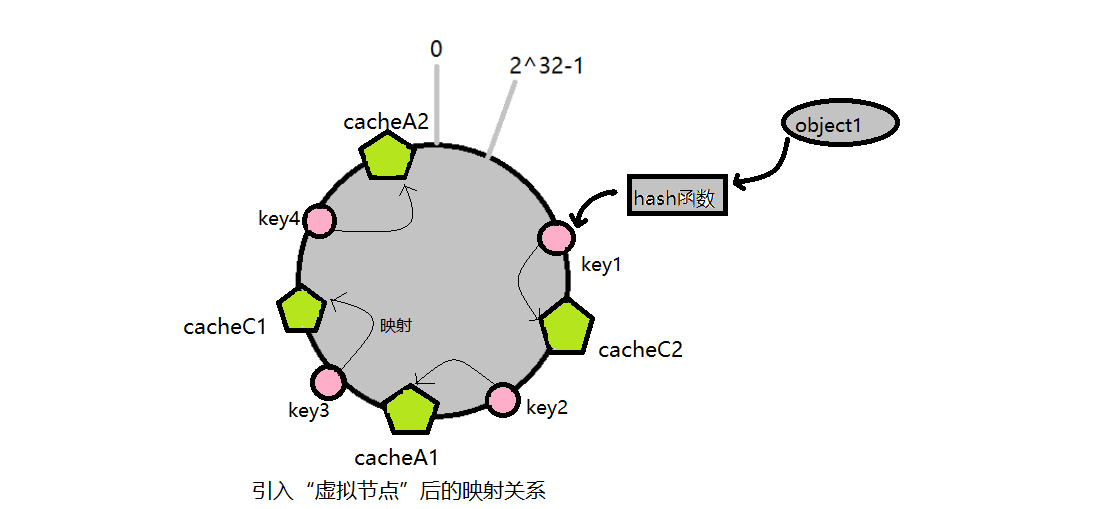objec1->cache C2 ； objec2->cache A1 ； objec3->cache C1 ； objec4->cache A2 ；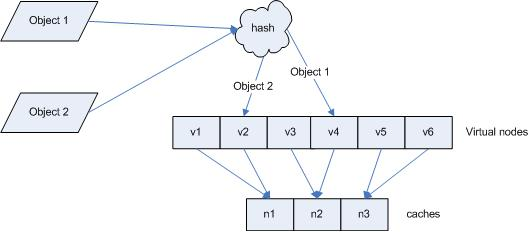“虚拟节点”的 hash 计算可以采用对应节点的 IP 地址加数字后缀的方式。例如假设 cache A 的 IP 地址为 202.168.14.241 。

Hash(“202.168.14.241”);

Hash(“202.168.14.241#1”); // cache A1

Hash(“202.168.14.241#2”); // cache A2

## 一个PHP的例子：

<?php
class FlexiHash{
private $serverList = array(); //服务器列表 private$isSorted = false;  //服务器列表是否已经排过序

//hash函数
function mhash($key){$md5 = substr(md5($key)0,8);$seed = 31;
$hash = 0; for($i = 0;$i < 8;$i ++){
$hash =$hash * $seed + ord($md5{$i}); } return$hash & 0x7FFFFFFF;
}

//添加服务器
function addServer($server){$hash = $this->mhash($server);
if(!isset($this->serverList[$hash])){
$this->serverList[$hash] = $server; }$this->isSorted = false;
return true;
}
//移除服务器
function removeServer($server){$hash = $this->mhash($server);
if(isset($this->serverList[$hash])){
unset($this->serverList[$hash]);
}
$this->isSorted = false; return true; } //查找映射服务器（算法实现函数） function lookup($key){
$hash =$this->mhash($key); if(!$this->isSorted){
krsort($this->serverList,SORT_NUMERIC);$this->isSorted = true;
}
foreach($this->serverList as$pos => $server){ if($hash >= $pos){ return$server;
}
}
return reset(\$this->serverList);  //返回第一个元素
}
}